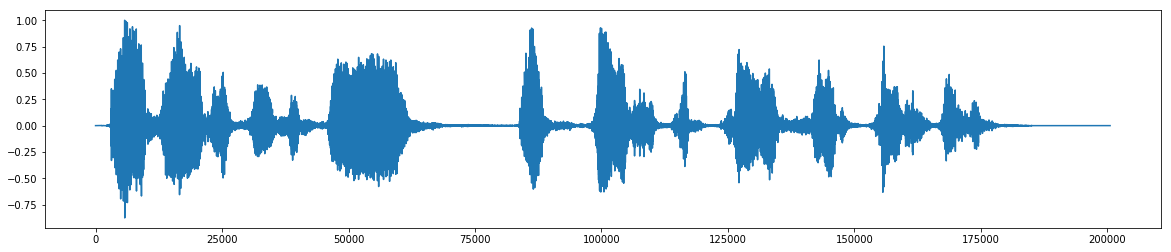# Noise reduction using spectral gating in python

Posted on Sat 07 July 2018 in Signal Processing

A pip installable package for this code is now available at https://github.com/timsainb/noisereduce

### Noise reduction in python using¶

• This algorithm is based (but not completely reproducing) on the one outlined by Audacity for the noise reduction effect (Link to C++ code)
• The algorithm requires two inputs:
1. A noise audio clip comtaining prototypical noise of the audio clip
2. A signal audio clip containing the signal and the noise intended to be removed

#### Steps of algorithm¶

1. An FFT is calculated over the noise audio clip
2. Statistics are calculated over FFT of the the noise (in frequency)
3. A threshold is calculated based upon the statistics of the noise (and the desired sensitivity of the algorithm)
4. An FFT is calculated over the signal
5. A mask is determined by comparing the signal FFT to the threshold
6. The mask is smoothed with a filter over frequency and time
7. The mask is appled to the FFT of the signal, and is inverted
In :
import IPython
from scipy.io import wavfile
import scipy.signal
import numpy as np
import matplotlib.pyplot as plt
import librosa
%matplotlib inline


In :
wav_loc = "assets/audio/fish.wav"
data = data / 32768

In :
# from https://stackoverflow.com/questions/33933842/how-to-generate-noise-in-frequency-range-with-numpy
def fftnoise(f):
f = np.array(f, dtype="complex")
Np = (len(f) - 1) // 2
phases = np.random.rand(Np) * 2 * np.pi
phases = np.cos(phases) + 1j * np.sin(phases)
f[1 : Np + 1] *= phases
f[-1 : -1 - Np : -1] = np.conj(f[1 : Np + 1])
return np.fft.ifft(f).real

def band_limited_noise(min_freq, max_freq, samples=1024, samplerate=1):
freqs = np.abs(np.fft.fftfreq(samples, 1 / samplerate))
f = np.zeros(samples)
f[np.logical_and(freqs >= min_freq, freqs <= max_freq)] = 1
return fftnoise(f)

In :
IPython.display.Audio(data=data, rate=rate)

Out:
In :
fig, ax = plt.subplots(figsize=(20,4))
ax.plot(data)

Out:
[<matplotlib.lines.Line2D at 0x7f67290bac50>]noise_len = 2 # seconds

fig, ax = plt.subplots(figsize=(20,4))